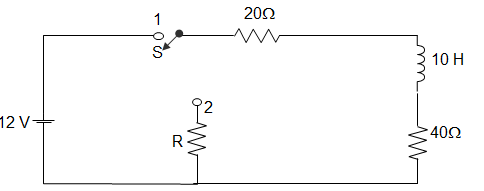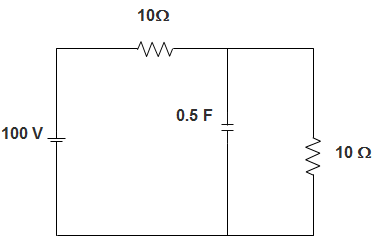Q.1
##### A coil is designed for high Q performance at a rated voltage and a specified frequency. If the frequency is made twice the original and the coil is operated at the same rated voltage, then the Q factor will be affected as ____________• a) Q is halved
• b) Q remains unchanged
• c) Q is doubled
• d) Q increases or decreases but magnitude cannot be measured
Q.2
##### A coil is designed for high Q performance at a rated voltage and a specified frequency. If the frequency is made twice the original and the coil is operated at the same rated voltage, then the active power P will be affected as ____________
• a) P is halved
• b) P remains unchanged
• c) P is doubled
• d) P decreases 4 times
Q.3
• a) 9 H
• b) 21 H
• c) 11 H
• d) 6 H
Q.4
• a) 10.56
• b) -28.57
• c) 11.80
• d) -11.80
Q.5
##### The CT supplies current to the current coil of a wattmeter power factor meter, energy meter and, an ammeter. These are connected as?• a) All coils in parallel
• b) All coils in series
• c) Series-parallel connection with two in each arm
• d) Series-parallel connection with one in each arm
Q.6
• a) 35
• b) 37.24
• c) 45
• d) 50.25
Q.7